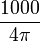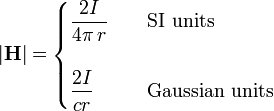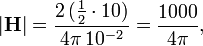# Oersted (unit)

In physics, the oersted (symbol Oe) is the unit of magnetic field strength |H| in the emu (electromagnetic unit) and Gaussian systems of units. Both systems are cgs (centimeter-gram-second) systems.

The relation to the corresponding SI unit (ampere times turn per meter) is

1 Oe =$\frac{1000}{4\pi}$ A⋅turn/m.

See solenoid for the explanation of the SI unit, which is one of the few electromagnetic units that carries no name.

The oersted is named for the Danish scientist Hans Christian Oersted.

## Definition

The definition of Oe follows from the Biot-Savart law giving the magnetic field strength field |H| due to an electric current I in an infinitely long straight conductor,$|\mathbf{H}| = \begin{cases} \frac{2I}{4\pi\, r}}& \quad \hbox{SI units} \\ \\ \frac{2I}{c r}}& \quad \hbox{Gaussian units}\\ \end{cases}$

where r is the distance of the field point to the conductor and c is the speed of light (≈ 3⋅1010 cm/s). In the SI definition r is in meters, whereas in Gaussian units r is in centimeters.

For Gaussian units, the oersted is defined as the strength of the magnetic field at a distance of 1 centimeter from a straight conductor of infinite length and negligible circular cross section that carries a current of ½⋅c statA (statampere).

## Conversion to SI units

Because the speed of light c in two different units is needed, it will be written numerically—but approximately—as 3·108 m/s (SI) or 3·1010 cm/s (cgs). Since only the ratio (exactly 100) is needed, the end result is exact.

In order to explain the factor 1000/4π, relating Oe (Gaussian) and A⋅turn/m (SI), consider an infinite wire carrying a current of ½⋅3⋅1010  statA and measure |H| at one cm distance from the wire. By definition the field has the strength 1 Oe. Noting that 1 statA = 1 A/(10⋅c), the current is equal to

I = ½⋅3⋅1010 statA = ½⋅3⋅1010 A/(3⋅109) = ½⋅10 A,

Applying the Biot-Savart equation valid in SI units (current I in A, distance r in m), we find that the field in the same physical setup, but expressed in A⋅turn/m is$|\mathbf{H}| = \frac{2 \,(\tfrac{1}{2}\cdot 10)}{4\pi \, 10^{-2}} = \frac{1000}{4\pi},$

from which follows that a field of strength 1 Oe has strength$\tfrac{1000}{4\pi}$  A·turn/m exactly. Since "turn" (number of turns) is a dimensionless number, it is often omitted in the SI unit, which then becomes simply A/m.